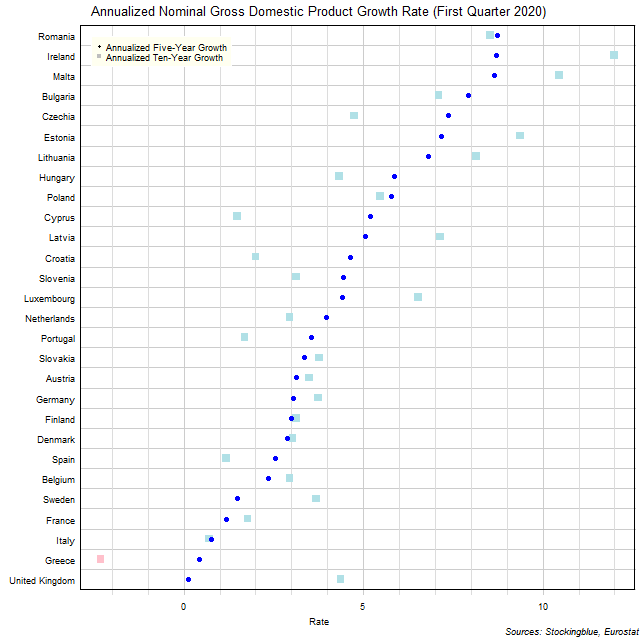# Long-Term EU GDP Growth Rate by State, Fourth Quarter 2019

Sep 17, 2020The chart above shows the annualized nominal gross domestic product (GDP) growth rate in each EU state over the past five years as of the fourth quarter of 2019 and the growth rate over the past ten years.  Greece is the only state to experience negative growth over either period.

## Findings

• The difference between the state with the largest annualized five-year growth rate, Romania, and the state with the smallest, the United Kingdom, is 8.61 percentage points.
• The difference between the state with the largest annualized ten-year growth rate, Ireland, and the state with the smallest, Greece, is 14.30 percentage points.
• The median annualized five-year growth rate in the 28 EU states is 4.19% and the mean 4.37%.
• The median annualized ten-year growth rate in the 28 EU states is 3.71% and the mean 4.45%.
• Thirteen states' economies grew faster over the past five years than they did over the past ten years.  The remaining 15 states saw their economies grow slower over the past five years than they did over the past ten years.

## Caveats

• Data is from the fourth quarters of 2009, 2014, and 2019.
• The data is seasonally adjusted in current euros.
• The data is annualized by multiplying the quarterly figure by four.
• All figures are rounded to the nearest hundredth.

## Details

The United Kingdom had the worst performance over the past five years with an annualized growth rate of 0.12%.  Romania had the best performance with an annualized gain of 8.73%.

Over the past ten years, Greece had the worst performance with an annualized growth rate of -2.31% while Ireland had the best performance with an annualized growth rate of 11.99%.

The United Kingdom saw the worst change in its growth rate between its annualized ten-year growth and its annualized five-year growth slowing its rate by 4.25 percentage points.  Cyprus had the best change in its growth rate between its annualized ten-year growth and its annualized five-year growth ramping up its rate by 3.71 percentage points.

Cyprus went from having the 25th largest growth rate over the past ten years to the 10th largest growth rate over the past five years.  Conversely, the United Kingdom went from having the 11th highest growth rate over the past ten years to the 28th highest growth rate over the past five years.  Only three states had annualized growth rates in the top five both over the past ten years and over the past five years: Romania (4th highest ten-year, highest five-year), Ireland (highest annualized growth over ten years, 2nd highest growth over five years), and Malta (2nd highest ten-year, 3rd highest five-year).  Two states had growth rates in the bottom five both over both periods: Italy (27th ten-year, 26th five-year) and Greece (28th ten-year, 27th five year).

## Sources

Filed under: Charts and Graphs